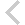FICO Xpress Optimization Examples Repository
 FICO Optimization Community FICO Xpress Optimization HomeEffects of convexity

Description
Nonlinear example demonstrating the effects of convexity

Further explanation of this example: 'Xpress NonLinear Reference Manual'

Source Files
By clicking on a file name, a preview is opened at the bottom of this page.

xnlp_convexity.mos

! Xpress-NonLinear example demonstrating the effects of convexity
!
! This example first solves a convex quadratic problem, for which Xpress-NonLinear
! find a proven optimal solution, then solves a non-covex one for which a local
! optimum is found.
!
! This example demonstrates a particular non-linear optimization concept as related
! to Xpress NonLinear.
! The version of the example is for Xpress 7.5.
!
!  (c) 2013 Fair Isaac Corporation
model mmxnlp_nlp_duals
uses "mmxnlp", "mmsystem";

! Scaling parameter for problem size. Please note, there is a quadratic response
! in terms of complexity
parameters
N = 500
end-parameters

! A function to translate the status of the solver ot text
public function nlpstatustext(val: integer): text
case val of
XNLP_STATUS_UNSTARTED: returned:= "NLP solving not started."
XNLP_STATUS_LOCALLY_OPTIMAL: returned:= "Local optimum found."
XNLP_STATUS_OPTIMAL: returned:= "Optimal solution found."
XNLP_STATUS_LOCALLY_INFEASIBLE: returned:= "NLP locally infeasible."
XNLP_STATUS_INFEASIBLE: returned:= "Problem is infeasible."
XNLP_STATUS_UNBOUNDED: returned:= "Problem is unbounded."
XNLP_STATUS_UNFINISHED: returned:= "NLP solving unfinished."
XNLP_STATUS_UNSOLVED: returned:= "NLP unsolved due to numerical issues."
end-case
end-function

declarations
R   = 1..N
x   : array(R) of mpvar
z,t : mpvar
end-declarations

! Switch logging on to see the effect of convexity related to the automatic solver
! selection
! setparam("xnlp_verbose",true)

! set the random seed for reproducablity
setrandseed(123)

! Create a large convex QCQP
Constraint := sum(i in R, j in i..N) random*(x(i)-random)*(x(j)-random) +
sum(i in R) N*x(i)^2 <= t
sum(i in R) random^2*x(i)^2 <= t
sum(i in 2..N-1) (random*x(i-1) + random*x(i) + random*x(i+1)) <= t
forall(i in 2..N-1) (random*x(i-1) + random*x(i) + random*x(i+1)) <= t

! The base problem is a convex quadratic constrained problems, it will be solved
! using the purpose written barrier solver
z <= 1
z >= 0
writeln("Solving a convex quadratic problem: ")
minimize(t^2+z^2)
writeln("  solver returned status = ",nlpstatustext(getparam("XNLP_STATUS")))

! This is essentially an equivalent, but no longer convex problem, it will be
! directed to Knitro
! No longer using a purpose built solver makes the solve more expensive
writeln("Solving a non-convex quadratic problem: ")
minimize(t^2-z^2)
writeln("  solver returned status = ",nlpstatustext(getparam("XNLP_STATUS")))

end-model